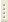06.02.2023Physics of Мetals

Prof. M.R.Trunin

The course embraces: early Drude-Sommerfeld theory, electron energy bands, Fermi surface and elementary calculation techniques; kinetic properties: electrical and galvanomagnetic effects; scattering processes; behavior of metals in high frequency fields; quantum conductivity effects; electromagnetic wave propagation in metals in presence of magnetic field.

Curriculum

1. General concepts of metal theory. Concept of free electron model, mean free path. Assumptions of Drude theory. Statistic electrical conduction in metals. High frequency conductivity. Thermal conduction of metals. Wiedemann-Franz law.
2. Thermodynamic properties of free electron gas. Ground state. Application of Fermi-Dirac distribution. Thermal capacity of electron gas. Sommerfeld theory of metal conductivity.
3. Crystal lattice. Unit cell. Reciprocal lattice. Brillouin bands. Normal modes and phonons. Thermal capacity of lattice. Debye and Einstein models. Thermal expansion of metals.
4. Band theory. Periodic potential. Bloch theorem. General properties of electron energy spectrum in metals. Electrons in weak periodic potential.
5. Strong coupling method. Diagrams of expanded, reduced and recurrent bands. Metals, semiconductors, dielectrics. Harrison plotting of Fermi surface.
6. Quasiclassical model of electron dynamics. Equation of motion in electric and magnetic field. Electrons and holes. Effective mass. Cyclotron mass. Motion trajectory in magnetic field. Trajectory types.
7. Kinetic equation. Collision integral. Relaxation time approximation. Conductivity in stationary electric field. Fermi surface shift.
8. Scattering cross-section and mean free path. Scattering on neutral and charged impurities, dislocations, phonons. Electron-electron scattering. Combination of scattering processes.
9. Magnetoresistance. Conductivity tensor in zero and first approximations. Resistance tensor. Hall effect. Magnetoresistance in two-band model. Effect of Fermi surface shape on electrical resistance. Open trajectory role.
10.  Density of states. Electrons in strong magnetic field. Quasiclassical quantization. Landau levels. Bloch electron level degeneracy. Oscillations of thermodynamic values. De Haas-van Alphen and Shubnikov-De Haas effects.
11.  Normal skin-effect. Leontovich boundary conditions and metal surface impedance. Abnormal skin-effect. Inefficiency concept. Chambers formula.
12.  Cyclotron resonance. Cyclotron resonance orbit cutoff. Rf size effects.
13.  Skip orbits. Magnetic surface levels. Interference effects in magnetic field. Conductivity quantum correction.
14.  Electromagnetic wave damping mechanisms in metals. Landau damping. Wave propagation in presence of magnetic field. Types of wave: helicon, Alfvén and cyclotron waves in metals.